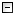Home Control Panel Login Register Search Submit Logout About me Contact meMy ResearchWeb This Site
 Home > My Research > SAS GCM power
 Category: SAS GCM power Below are collections of SAS macros to calculate power for growth curve analysis with/without missing data. These macros are licensed under the GNL General Public License Version 2.0. You can use/modify/distribute those macros. For questions or comments, please contact Johnny Zhang at zhiyongzhang(@)nd.edu. It would be appreciated if you cite the macros in the following way:Zhang, Z., & Wang, L. (2009). Power analysis for growth curve models using SAS. Behavior Research Methods, 41(4), 1083-1094. Request a copy Download a copy The complete simulation results for Zhang & Wang (2009) can be found here Power plot final results online.htm.

Contents
 Here is a list of software/programs we were aware of that can conduct power anlaysis for growth curve models/multilevel models.
 The SAS macros estimate the power for testing nonlinear trajectory using the latent basis growth curve model. The macros ultilize both PROC MIXED and PROC NLMIXED to estimate statistical power.
 The SAS macros estimate the power of testing whether there is a quadratic term in the model by comparing a quadratic growth curve model and a linear growth curve model.
 The macros estimate the power for a conditional linear growth curve model where the individual rates of change are predicted by the group information (covariates). The macros can be used to estimate the power of detecting the treatment effects.
 The macros estimate the power for evaluting parameters in an exponential growth curve model. R=10000 are recommended. In this example, the mean change between the iniital status and the asymptotic level is tested. It is recommended that a single replication of data is first tested to observe the behavior of convergence. The PROC NLMIXED is used here. The power estimation for the exponential growth curve model can be very slow.
 The macros estimate the power for evaluating the average rate of change. When there are missing data, R=5000 are recommended. The PROC MIXED is used to estimate the model parameters.
 Total 6 ,Display 1 - 6 1
 Copyright © 2003-13 Zhiyong Zhang \'s Psychometric WebsiteMaintained by Zhiyong Zhang